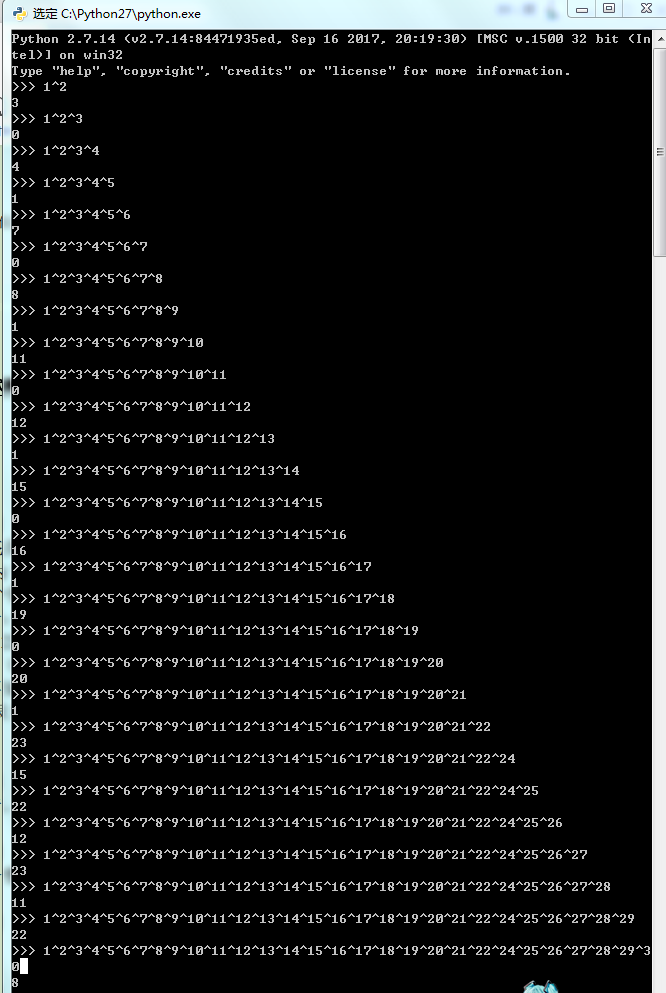# T1 取石子游戏

2

2

3

1

0

### 【样例解释】

$n=2$ 时小林只有一种取胜方式，即取在第二堆石子中取一粒。$n=3$时小林必败，因此输出 0。

### 【数据规模】1 n % 4=1
n+1 n % 4=2
0 n % 4=3
n n % 4=0

n % 4=0 或 2 时，为了使异或序列的结果为零。n+1和n的位数一样，设它们的 最高位为 t，n/n+1 的第 t 位为 1 ，为使n/n+1 变为 0 ，必须修改一个第 t 位为 1 的数。显然修改任一第 t 位为 1 的数都可以使 n/n+1变为 0 。答案即为 n 去掉最高位再加 1 。

/*我HUI就是饿死*/
/*死外边儿*/
/*在这跳下去*/
/*也不会敲高精去拿那十分*/
/*↓↓↓*/
/*切了T1真好*/

/*极力掩盖我不会高精的事实*/
#include<cmath>
#include<string>
#include<cstdio>
#include<cstring>
#include<iostream>
#include<algorithm>
#define ll long long
#define IL inline
#define R register
using namespace std;
ll n,T,x,y;

int f=1;x=0;char s=getchar();
while(s>'9'||s<'0'){if(s=='-')f=-1;s=getchar();}
while(s<='9'&&s>='0'){x=x*10+s-'0';s=getchar();}
x*=f;
}
inline void print(ll x){
if(x<0){
putchar('-');
x=-x;
}
if(x>9)	print(x/10);
putchar(x%10+'0');
}

int main()
{
freopen("nim.in","r",stdin);
freopen("nim.out","w",stdout);
while(T--){
if(n%4==3){
print(0);
cout<<endl;
goto chui_zi_most_grass_egg;
}
if(n%4==1){
print((n+1)/2);
cout<<endl;
goto chui_zi_most_grass_egg;
}
if(n%4==2){
x=n;
y=1;
while(y<=x){
y*=2;
}
y /= 2;
x-=y;
x++;
print(x);
cout<<endl;
goto chui_zi_most_grass_egg;
}
if(n%4==0){
x=n;
y=1;

while(y<=x){
y*=2;
}
y /= 2;
x-=y;
x++;
print(x);
cout<<endl;
goto chui_zi_most_grass_egg;
}
chui_zi_most_grass_egg:;
}
return 0;
}

posted @ 2018-09-25 11:03  enceladus  阅读(164)  评论(0编辑  收藏